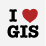# Find best order route is slow

2082
7
01-14-2016 02:09 AMRegular Contributor II

We have a network dataset with around 300k lines.

We have a car that has to do inspections on some elements along a route. It needs to visit 500-700 elements (most of them are close to each other).

The points create one simple route but are not ordered.

The way we solve the problem is to load the points as steps and to ask for best order route (usually the first point is really the start).

It creates a good route that visits every element.

The only problem is the time it takes. About 7 minutes to 700 points.

When the points is ordered it takes a few seconds.

Is there any way to speed the best route algorithm?

Thanks

Tags (3)
7 RepliesbyMVP Esteemed Contributor

That is a lot of combination to figure out.  Have you tried a

lexicographic sort on the coordinates? Or is that what you mean by sorted? You really need to limit you search radius for the next possible candidate.Regular Contributor II

Hi Dan

The route is not going in one direction only but it might be a good idea to order the points by ariel distance first and then run the route in this order.

Do you know any ready to use tool that will order the point (starting from a known point) or do I have to write it?

ThanksbyMVP Esteemed Contributor

short of a simple sort on the XY coordinates of the points, which may speed things up, a spanning tree from an origin point may help since it provides the shortest connections between points.  I haven't experimented in a long time, but you could give this a try http://www.arcgis.com/home/item.html?id=6ce9db93533345e49350d30a07fc913a (spanning tree tools) it may give you some ideas.byMVP Frequent Contributor

Mody, NA based on the well-known Dijkstra's algorithm so if you need speed you need more rules/restriction so algorithm discards useless path evaluatebyMVP Frequent Contributor

Mody you can also approximate solution collapse your points of same edge in a single point ('most of them are close to each other') and then order all points after solve.byMVP Esteemed Contributor

I think Domenico is on to something...your problem could be greatly simplified if you could group points or cluster them and use a representative location.  If you have 5 origin points all on the same block, it really doesn't matter their individual route solutions, since they will effectively be identical...if you throw a coffee break into the mix, your grouping distance could expand to a couple of blocks.  So I would follow his suggestion and have a good look at your point pattern(s) and see if there is any inherent clustering as origins and/or destinationsRegular Contributor II

Hi

I think I found a great solution (Dan post gave me the idea).

We work so much with complex software that we forget how quick a computer is when it just do in memory calculation.

The code below sort the point by distance (direct distance). It is running in memory and takes less the 3 seconds for 2000 points (including reading and writing to layer).

`import arcpyimport sysimport tracebackimport mathpoints = arcpy.GetParameterAsText(0)# globalscoords = []order = []# find the closest point that is not connecteddef FindClosest(x,y):    global coords    global order    max1 = 1000000000000    closest = -1    for i in range(0, len(order)):        if (order != 0): continue        # noo need for sqrt - just looking for the smallest        dist = math.pow((coords - x),2) + math.pow((coords - y),2)        if (dist < max1):            max1 = dist            closest = i    return closesttry:    #read into memory    with arcpy.da.SearchCursor(points, ["SHAPE@X", "SHAPE@Y"]) as cursor:        for row in cursor:            p = [row,row]            coords.append(p)            order.append(0)    arcpy.AddMessage("read %s records" % len(coords))    # first record    next1 = 2    order = 1    x1 = coords    y1 = coords    # endless loop until we have no free points    while(True):        x = FindClosest(x1,y1)        if(x == -1): break # done        order = next1        x1 = coords        y1 = coords        next1 = next1 + 1    # update results    i = 0    with arcpy.da.UpdateCursor(points, ["order1"]) as cursor:        for row in cursor:            row = order            cursor.updateRow(row)            i = i + 1    #for i in range(0,len(order)):    #    arcpy.AddMessage("point %s order = %s" % (order,i))except:`